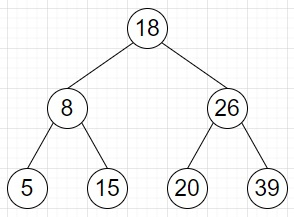# Check if an array represents Inorder of Binary Search tree or not in Python

Suppose we have an array of numbers called nums. We have to check whether the array is holding elements of a binary search tree in the sequence of its inorder traversal or not.

So, if the input is like nums = [5, 8, 15, 18, 20, 26, 39], then the output will be True as this is inorder traversal ofTo solve this, we will follow these steps −

• size := size of nums
• if size either 0 or 1, then
• return True
• for i in range 1 to size - 1, do
• if nums[i - 1] > nums[i], then
• return False
• return True

Let us see the following implementation to get better understanding −

## Example

Live Demo

def solve(nums):
size = len(nums)
if size == 0 or size == 1:
return True
for i in range(1, size):
if nums[i - 1] > nums[i]:
return False
return True
nums = [5, 8, 15, 18, 20, 26, 39]
print(solve(nums))

## Input

[5, 8, 15, 18, 20, 26, 39]

## Output

True